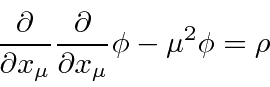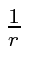## Classical Field Theory

A review of classical field theory is useful to ground our development of relativistic quantum field theories for photons and electrons. We will work with 4-vectors like the coordinate vector belowusing theto get ain the time term in a dot product (instead of using a metric tensor).

A Lorentz scalar Lagrangian density will be derived for each field theory we construct. From the Lagrangian we can derive a field equation called the Euler-Lagrange equation.The Lagrangian for a massive scalar fieldcan be deduced from the requirement that it be a scalarwhere the last term is the interaction with a source. The Euler-Lagrange equation giveswhich is the known as the Klein-Gordon equation with a source and is a reasonable relativistic equation for a scalar field.

Using Fourier transforms, the field from a point source can be computed.This is a field that falls off much faster than. A massive scalar field falls off exponentially and the larger the mass, the faster the fall off. This fits the form of the force between nucleons fairly well although the actual nuclear force needs a much more detailed study.

Jim Branson 2013-04-22